#### Thank you for registering.

One of our academic counsellors will contact you within 1 working day.

Click to Chat

1800-5470-145

+91 7353221155

CART 0

• 0
MY CART (5)

Use Coupon: CART20 and get 20% off on all online Study Material

ITEM
DETAILS
MRP
DISCOUNT
FINAL PRICE
Total Price: Rs.

There are no items in this cart.
Continue Shopping

# Chapter 6: Trigonometric Identities Exercise – 6.2

### Question: 1

If cos θ = 4/5, find all other trigonometric ratios of angle θ.

### Solution:

We have: sin θ = 1 - cos2θ= 3/5

Therefore, sin θ = 3/5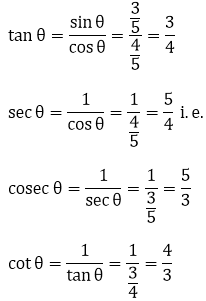### Question: 2

If sin θ = 1/√2, find all other trigonometric ratios of angle θ.

### Solution:

We have,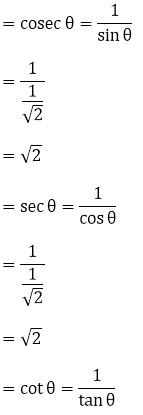=1/1

=1

### Question: 3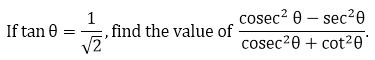### Solution:

We know that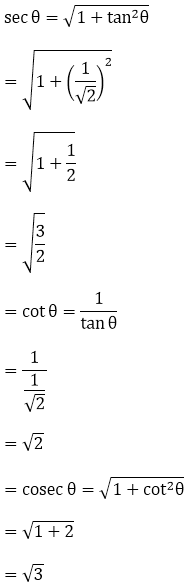Substituting it in equation (1) we get### Question: 4### Solution:

We know that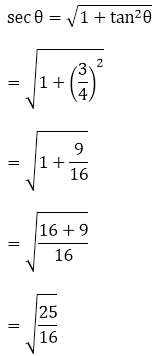sec θ = 5/4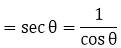= 1/(5/4)

= 4/5

= cos θ

Therefore, We get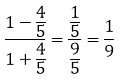### Question: 5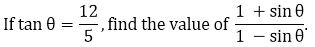### Solution: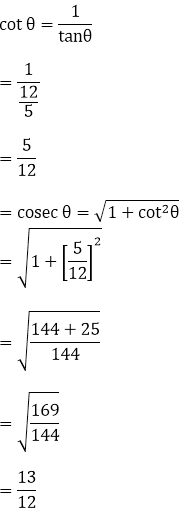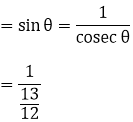= 12/13

i. e. We get= 25/1

= 25.

### Question: 6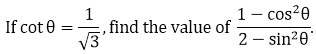### Solution:= cos θ = sin θ × cot θ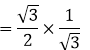= 1/2

Therefore, on substituting we get= 3/5.

### Question: 7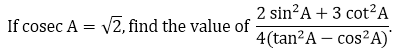### Solution:

We know that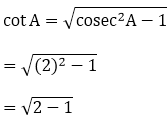= 1.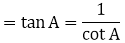= 1/1

= 1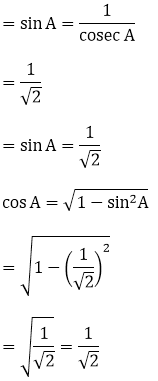On substituting we get:= 4/2

= 2

### Question: 8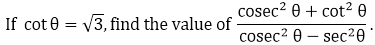### Solution: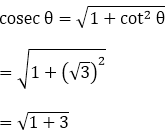= 2cos θ = cot θ. sin θ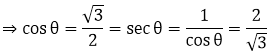On substituting we get:= 21/8

### Question: 9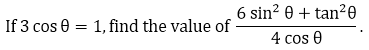### Solution: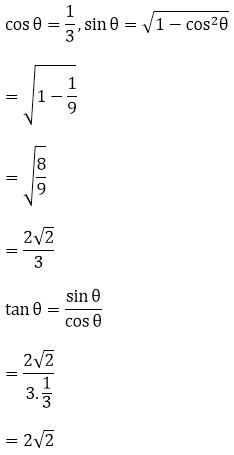On substituting we get= 40/4

= 10

### Question: 10

If √3 tan θ = sin θ, find the value of sin2θ - cos2θ.

### Solution: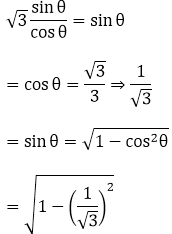= sin2θ - cos2θ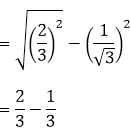= 1/3

### Question: 11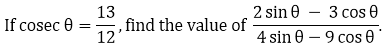### Solution: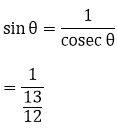= 12/13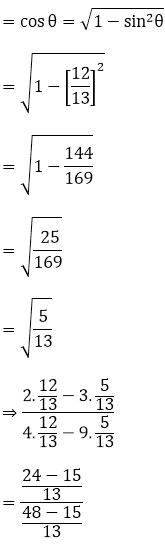= 9/3

= 3

### Question: 12

If sin θ + cos θ =√2 cos(90°- θ), find cot θ.

### Solution:

= sin θ + cos θ = √2 sin θ [cos (90 - θ) = sin θ]

⇒ cos θ = √2 sin θ – sin θ

⇒ cos θ = sin θ (√2 - 1)

Divide both sides with sin θ we get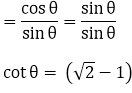= √2 - 1.

### Question: 13

If 2 sin2 θ − cos2θ = 2, then find the value of θ.

### Solution:

2 sin2 θ − cos2 θ = 2

⇒ 2 sin2 θ − (1 − sin2 θ) = 2

⇒ 2 sin2 θ − 1 + sin2 θ = 2

⇒ 3 sin2 θ = 3

⇒ sin2 θ = 1

⇒ sin θ = 1

⇒ sin θ =  sin 90°

⇒ θ = 90°

### Question: 14

If √3 tan θ – 1 = 0, find the value of sin2 θ - cos2 θ.

### Solution:√3 tan θ = tan 30° θ = 30°

Now, sin2θ - cos2 θ = sin2 (30°) - cos2 (30°)